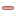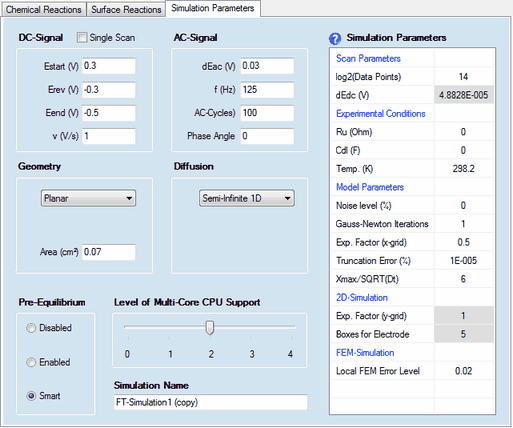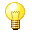﻿ Ribbon Bar Command: Edit
DigiElch Help

# Ribbon Bar Command: Edit# Ribbon Bar Command: EditThis command opens a FT-Property Dialog consisting of 3 property pages:Property Page: Chemical Reactions
 The Property Page: Chemical Reactions works as previously described for the CV-Simulation Document.Property Page: Surface Reactions
 The Property Page: Surface Reactions works as previously described for the CV-Simulation Document.Property Page:  Simulation ParametersA value of Cdl (F) written in magenta indicates that a time/potential dependent double layer capacity has been entered by the user. The polynomial coefficients describing the dependence of the double layer capacity as function of the electrode potential can be edited by clicking with the right mouse button while the cursor is localized over the input field associated with Cdl (F).

The following parameter groups

Geometry

Diffusion

Pre-Equilibrium

Level of Multi-Core CPU Support

Simulation Name

as  well as the Simulation Parameters headlined

Experimental Conditions

Model Parameters

2D-Simulation

FEM-Simulation

work exactly in the same way as already described for cyclic voltammetry.

## 1. DC - Signal

Check Box: Single Scan
Activate this check box if only the forward scan of the DC-signal needs to be simulated. Eend (V) is automatically set equal to Erev(V) and the input field for Eend (V) will be blocked.

Estart (V), Erev, Eend (V), v(s)
The DC-scan can be composed of up to 2 scan segments characterized by starting potential, Estart (V), end-potential, Eend (V), and scan rate, v (V/s).

## 2. Scan Parameters

log2(Data Points)
The number of data points used for generating the signal. It must be an integer power of 2 and is 2^14 = 16384 by default.

dEdc (V)
The potential difference between subsequent data points (fitting the selected number of Data Points) is shown in the (read only) parameter dEdc(V).

## 3. AC - Signal

dEac (V), f(Hz), Phase Angle
The superimposed AC-signal is characterized by Amplitude, dEac (V), frequency, f(Hz), and Phase Angle. The frequency is adjusted such as to ensure an integer value for the number of AC-Cycles.

AC-Cycles
The integer number of AC-Cycles which is superimposed to the DC-signal.